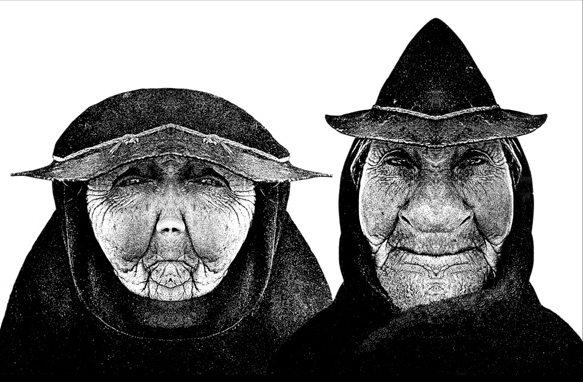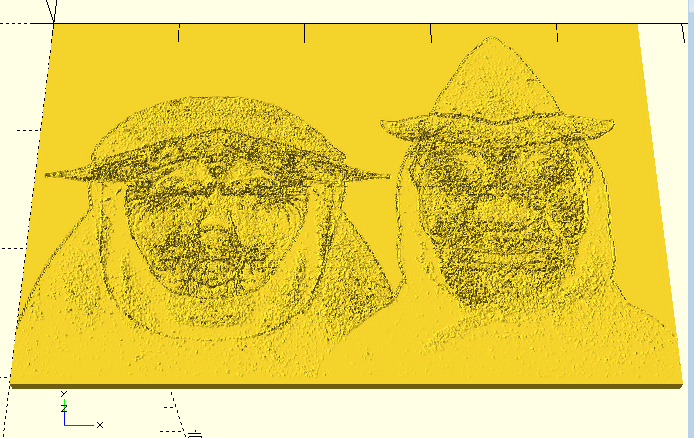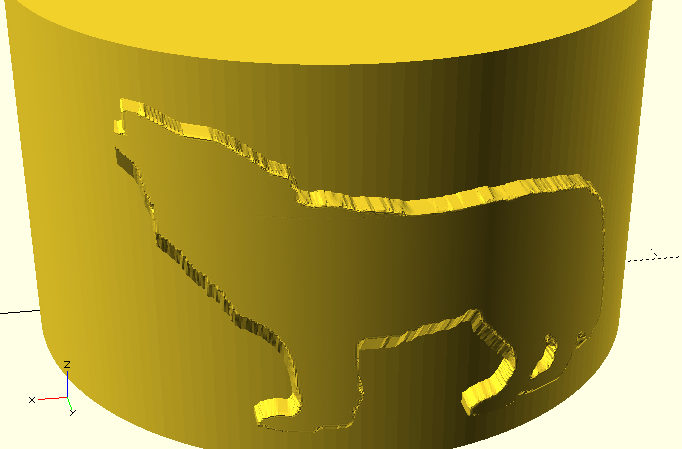# AngelScript CSG – part 3

Download AngelScript CSG version V2.0-02, with IDE included
Windows 64bit here.
Linux (K)ubuntu 15.10 64bit here.

This is an update to previous articles part 1 and part 2 on AngelScript CSG, which is using AngelScript as a language for Constructive Solid geometry (CSG).

Today's update is to describe the latest couple of changes. One may now create polyhedrons from image files and from OpenSCAD style text files. To support images a new command “image2d” has been introduced, supporting image files of type PNG, JPG, GIF, XPM and more. It is also possible to resample an imported image before using it to create a 3d model.Ektepar (Carsten J. Arnholm) - Copyright ©From photo to 3d-model

The code:

 shape@ main_shape(){  ​​​​  ​​ ​​​​ image2d@ image = image2d("ektepar_ca.png"); ​​ ​​​​ uint w = image.width(); ​​ ​​​​ uint h = image.height(); ​​ ​​​​ double s =0.002; ​​ ​​​​ double dx=400; ​​ ​​​​ double dy=400; ​​ ​​​​ auto surface = loft3d( image,dx,dy,w); ​​ ​​​​ return ​​ mirror(0,1,0)* ​​ ​​ ​​ ​​ ​​ ​​ ​​ ​​ ​​ ​​​​ scale(s/5,s/5,2*s)*    ​​​​ polyhedron(surface,vec3d(0,0,-300),h);}

If the image is very large it is a good idea to downsize it before it is imported, or alternatively use the “resize” method supported on image2d objects. A third way is to keep the image size but reduce the final segmentation. To reduce the segmentation by half in the example above, you could enter w/2 in the call to loft3d and h/2 in the call to polyhedron.
Another example is using an
OpenSCAD surface text file to create a model of a wolf on a mug:Wolf from text file

As in the case with the image, the data is used to create a lofted surface and from that a polyhedron is created. In this case the data file contains 300 data points in X and 275 rowx (y).

 const double pi = 4.0*atan(1.0); double to_rad(double deg) { return pi*deg/180.0; } ​​ // from degree to radian shape@ main_shape(){  ​​​​  ​​ ​​​​ double h = 30; ​​ ​​​​ double r = 30; ​​ ​​​​ double e = 3;  ​​ ​​​​ solid@ cyl = cylinder(h+10,r+e);  ​​ ​​​​ // model space distance between the data points ​​ ​​​​ double dx=1; ​​ ​​​​ double dy=1;  ​​ ​​​​ // specify that default segmentation shall be used, i.e. ​​ ​​​​ // all points in the file in s-dir (=x) and t-dir (=y) ​​ ​​​​ int ns_seg = -1; ​​ ​​​​ int nt_seg = -1; ​​ ​​​​ loft3d@ surface = loft3d("model.data.txt",dx,dy,ns_seg);  ​​ ​​​​ solid@ ​​ wolf = translate(-23.1,-0.5,h+5) * ​​ ​​ ​​ ​​ ​​ ​​ ​​ ​​ ​​ ​​ ​​ ​​ ​​ ​​ ​​ ​​ ​​​​ rotate_x(to_rad(-90)) *  ​​ ​​ ​​ ​​ ​​ ​​ ​​ ​​ ​​ ​​ ​​ ​​ ​​ ​​ ​​ ​​ ​​​​ scale(0.154, 0.109, 1)* ​​ ​​ ​​ ​​ ​​ ​​ ​​ ​​ ​​ ​​ ​​ ​​ ​​ ​​ ​​ ​​ ​​​​ polyhedron(surface,vec3d(0,0,-30),nt_seg);  ​​​​  ​​ ​​​​ return union3d(cyl,wolf);} void main(){ ​​ ​​​​ shape@ obj = main_shape(); ​​ ​​​​ obj.write_csg(GetOutputFullPath('.csg')); }

# AngelScript CSG and OpenSCAD

Below is an updated list of the revised CSG related commands. For even more details, and documentation of the functions each object type provides, run the following command, where <filename> is your own AngelScript CSG file, or one of the provided samples.

> as_csg -doc <filename>.as

This will generate a file 'angelscript_csg_doc.txt' listing all the constructors and functions for all the command types.

 Angelscript CSG OpenSCAD 2d utilities pos2d  ​​ ​​​​ (position in 2d space) array type [x,y] spline2d (spline curve in 2d space) N/A image2d ​​ (bitmap image support) 'file' in surface command 2d primitives shape2d ​​ (abstract type) N/A circle(double r); circle(radius) square(double size, bool center=false); square(size,center) rectangle(double dx, double dy, bool center=false); square([width,height],center) polygon(array points); polygon([points]) polygon(pos2d@ p1,...); 2d booleans union2d(array shapes); union() { ... } ​​ union2d(shape2d@ shape, …); difference2d(array shapes); difference() { ... } difference2d(shape2d@ shape, …); intersection2d(array shapes); intersection() { ... } intersection2d(shape2d@ shape, …); hull2d(array shapes); hull() {...} hull2d(shape2d@ shape, …); minkowski2d(array shapes); minkowski() {...} minkowski2d(shape2d@ shape, …); offset2d(shape2d@ shape, double r); offset(r=...) soffset2d(shape2d@ shapes, double delta, bool chamfer=false); offset(delta=…, chamfer=false/true) 3d utilities pos3d  ​​ ​​​​ (position in 3d space) array type [x,y,z] vec3d  ​​ ​​​​ (vector in 3d space) array type [x,y,z] spline3d (spline curve in 3d space) N/A loft3d  ​​​​ (lofted surface) N/A 3d primitives solid  ​​​​ (abstract type) N/A cone(double h, double r); cylinder(h,r1,r2); cube(double size); cube(size); cuboid(double dx, double dy, double dz); cube([width,depth,height]); cylinder(double h, double r); cylinder(h,r); sphere(double r); sphere(r): polyhedron(points,faces)polyhedron(points,faces)polyhedron(surface,offset) polyhedron(points,faces) 3d booleans union3d(array shapes); union() { ... } ​​ union3d(solid@ shape, …); difference3d(array shapes); difference() { ... } difference3d(solid@ shape, …); intersection3d(array shapes); intersection() { ... } intersection3d(solid@ shape, …); hull3d(array shapes); hull() {...} hull3d(solid@ shape, …); minkowski3d(array shapes); minkowski() {...} minkowski3d(solid@ shape, …); linear_extrude(shape2d@ shape, double height); linear_extrude(height=..) { ... } rotate_extrude(shape2d@ shape, double angle); rotate_extrude(angle=..) { ... } 2d/3d transformations tmatrix  ​​​​ (abstract type) multimatrix([ …. ]); rotate_x(double rx); rotate([rx,0,0]); rotate_y(double ry); rotate([0,ry,0]); rotate_z(double rz); rotate([0,0,rz]); scale(double sx, double sy, double sz=1.0); scale([x,y,z]); translate(double dx, double dy, double dz=0.0); translate([dx,dy,dz]); mirror(double dx, double dy, double dz); mirror([dx,dy,dz]);